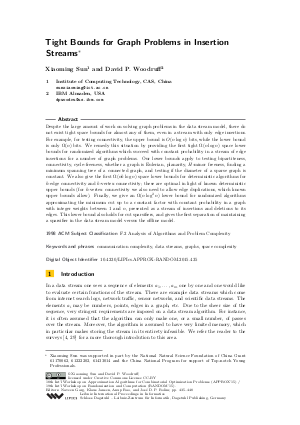Document# Tight Bounds for Graph Problems in Insertion Streams

### Authors Xiaoming Sun, David P. Woodruff## File

LIPIcs.APPROX-RANDOM.2015.435.pdf
• Filesize: 474 kB
• 14 pages

## Cite As

Xiaoming Sun and David P. Woodruff. Tight Bounds for Graph Problems in Insertion Streams. In Approximation, Randomization, and Combinatorial Optimization. Algorithms and Techniques (APPROX/RANDOM 2015). Leibniz International Proceedings in Informatics (LIPIcs), Volume 40, pp. 435-448, Schloss Dagstuhl - Leibniz-Zentrum für Informatik (2015)
https://doi.org/10.4230/LIPIcs.APPROX-RANDOM.2015.435

## Abstract

Despite the large amount of work on solving graph problems in the data stream model, there do not exist tight space bounds for almost any of them, even in a stream with only edge insertions. For example, for testing connectivity, the upper bound is O(n * log(n)) bits, while the lower bound is only Omega(n) bits. We remedy this situation by providing the first tight Omega(n * log(n)) space lower bounds for randomized algorithms which succeed with constant probability in a stream of edge insertions for a number of graph problems. Our lower bounds apply to testing bipartiteness, connectivity, cycle-freeness, whether a graph is Eulerian, planarity, H-minor freeness, finding a minimum spanning tree of a connected graph, and testing if the diameter of a sparse graph is constant. We also give the first Omega(n * k * log(n)) space lower bounds for deterministic algorithms for k-edge connectivity and k-vertex connectivity; these are optimal in light of known deterministic upper bounds (for k-vertex connectivity we also need to allow edge duplications, which known upper bounds allow). Finally, we give an Omega(n * log^2(n)) lower bound for randomized algorithms approximating the minimum cut up to a constant factor with constant probability in a graph with integer weights between 1 and n, presented as a stream of insertions and deletions to its edges. This lower bound also holds for cut sparsifiers, and gives the first separation of maintaining a sparsifier in the data stream model versus the offline model.
##### Keywords
• communication complexity
• data streams
• graphs
• space complexity

## Metrics

• Access Statistics
• Total Accesses (updated on a weekly basis)
0